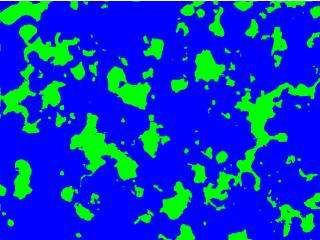-=+=- -=+=- -=+=- -=+=- -=+=- -=+=- -=+=- -=+=- -=+=- -=+=- -=+=- -=+=- -=+=- -=+=- -=+=- -=+=- -=+=- -=+=- -=+=- -=+=- -=+=- -=+=- -=+=- -=+=- -=+=- -=+=- -=+=- -=+=- -=+=- -=+=- (c) WidthPadding Industries 1987 0|570|0 -=+=- -=+=- -=+=- -=+=- -=+=- -=+=- -=+=- -=+=- -=+=- -=+=- -=+=- -=+=- -=+=- -=+=- -=+=- -=+=- -=+=- -=+=- -=+=- -=+=- -=+=- -=+=- -=+=- -=+=- -=+=- -=+=- -=+=- -=+=- -=+=- -=+=-

PakzBerzerk level generator

11th January 2022

Level generator from the classic game berzerk

PakzRecursive map generator

10th January 2022

[code]
-- Recursiveprocgen

-- Use this function to perform your initial setup
function setup()
print(''Hello World!'')
mw = 40
mh = 40
tw =*snip*

PakzL Systems - plant

28th October 2021

L systems - string with turtle instructions

Jayenkai20th August 2021

Wanting to grab all my ''Today's Stroll'' tweets and bundle them into a spreadsheet, I tried all manner of Web Rip tools, but nothing seemed to do what I wanted.

Pakzsprite sheet animator(bplus)

15th July 2021

A ancient tool to animate spritesheet

PakzRandom Walker Mazelike maps(Godot script)

16th February 2021

Some Godot script for creating maps.

PakzScanline fill

30th January 2021

Fast scanline fill routine in c language

DanPostfix evalution function.

12th September 2020

Hi,

this is my try at the postfix evaluation.

[code]
Global stackmax=100, stackpos=0, postfixmax=100, postpos=0, postitems=0, stackerror=0
Dim stack\$(stackmax)
Dim postfix\$(postfixmax)

;******* demo **********
Dim calc\$(10)

calc\$(00)=&qu*snip*

DanString byte packer/unpacker (crucher ?!)

29th February 2020

Pack/crunch a string, containing 2 bytes data

PakzOrientation of point vs line

24th November 2019

Is a point left or center or right of a line

PakzConvex Hull or Gift Wrapping algorithm

21st November 2019

create lines around set of points.

PakzPolygon vs Polygon Collision

13th November 2019

Is one polygon colliding with another polygon

PakzPoint versus Polygon Collision

2nd November 2019

Check if a point is inside a polygon

PakzShortest turn angle vs target angle

27th October 2019

Get distance between angle and target angle. (turrets/homing missiles)

HoboBenText wrapping in Go

16th October 2019

[code]
package main

import (
''strings''
)

// wrap quickly word-wraps a string
// (source: https://github.com/tawesoft/dialog/blob/master/wrap.go)
func wrap(message string, length int) string {
var atoms = strings.Fields(strings*snip*

PakzRandom Bag

16th July 2019

Get more predictable numbers

PakzRivers on a top down map.

30th May 2019

Create 2d Rivers

Pakz6th April 2019Create a greyscale stone like texture

PakzBitwise technique

23rd January 2019

Id System for neighbouring tiles

PakzMaps or oilish paintings

21st January 2019Smearing a additive brush

PakzPixel Art Pine Tree(s)

21st January 2019Generate sheets of pine trees(xmas?)

DanBitmapfont as text

18th November 2018

Bitmap fonts as easy as text. Color is changeable with the color statement.
This was made only for one font (at time), but can be expanded to use multiple fonts. For example, replacing img_font, img_char and Fontstring\$ for arrays.

This is for monospa*snip*

DanGetDrive\$ - create a list of available drive letters

2nd November 2018

Create a list of available drive letters

DanBlitz Eye's

1st November 2018

Now blitzbasic has eye's, too !

Dan